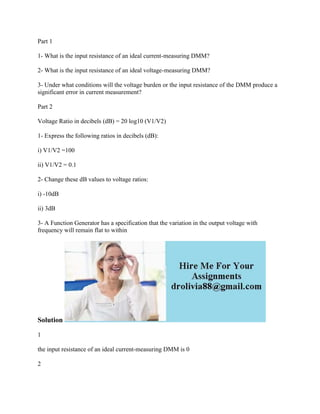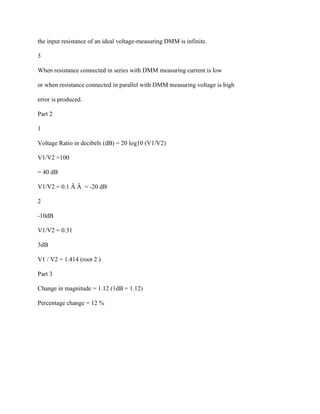Successfully reported this slideshow.

# Part 1 1- What is the input resistance of an ideal current-measuring D.docx×

# Part 1 1- What is the input resistance of an ideal current-measuring D.docx

Part 1
1- What is the input resistance of an ideal current-measuring DMM?
2- What is the input resistance of an ideal voltage-measuring DMM?
3- Under what conditions will the voltage burden or the input resistance of the DMM produce a significant error in current measurement?
Part 2
Voltage Ratio in decibels (dB) = 20 log10 (V1/V2)
1- Express the following ratios in decibels (dB):
i) V1/V2 =100
ii) V1/V2 = 0.1
2- Change these dB values to voltage ratios:
i) -10dB
ii) 3dB
3- A Function Generator has a specification that the variation in the output voltage with frequency will remain flat to within
Solution
1
the input resistance of an ideal current-measuring DMM is 0
2
the input resistance of an ideal voltage-measuring DMM is infinite.
3
When resistance connected in series with DMM measuring current is low
or when resistance connected in parallel with DMM measuring voltage is high
error is produced.
Part 2
1
Voltage Ratio in decibels (dB) = 20 log10 (V1/V2)
V1/V2 =100
= 40 dB
V1/V2 = 0.1 Â Â  = -20 dB
2
-10dB
V1/V2 = 0.31
3dB
V1 / V2 = 1.414 (root 2 )
Part 3
Change in magnitude = 1.12 (1dB = 1.12)
Percentage change = 12 %
.

Part 1
1- What is the input resistance of an ideal current-measuring DMM?
2- What is the input resistance of an ideal voltage-measuring DMM?
3- Under what conditions will the voltage burden or the input resistance of the DMM produce a significant error in current measurement?
Part 2
Voltage Ratio in decibels (dB) = 20 log10 (V1/V2)
1- Express the following ratios in decibels (dB):
i) V1/V2 =100
ii) V1/V2 = 0.1
2- Change these dB values to voltage ratios:
i) -10dB
ii) 3dB
3- A Function Generator has a specification that the variation in the output voltage with frequency will remain flat to within
Solution
1
the input resistance of an ideal current-measuring DMM is 0
2
the input resistance of an ideal voltage-measuring DMM is infinite.
3
When resistance connected in series with DMM measuring current is low
or when resistance connected in parallel with DMM measuring voltage is high
error is produced.
Part 2
1
Voltage Ratio in decibels (dB) = 20 log10 (V1/V2)
V1/V2 =100
= 40 dB
V1/V2 = 0.1 Â Â  = -20 dB
2
-10dB
V1/V2 = 0.31
3dB
V1 / V2 = 1.414 (root 2 )
Part 3
Change in magnitude = 1.12 (1dB = 1.12)
Percentage change = 12 %
.